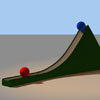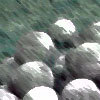#### You may also like### Whoosh

A ball whooshes down a slide and hits another ball which flies off the slide horizontally as a projectile. How far does it go?### Escape from Planet Earth

How fast would you have to throw a ball upwards so that it would never land?### Cannon Balls

How high will a ball taking a million seconds to fall travel?

# Angle of Shot

##### Age 16 to 18Challenge Level

When a projectile is fired, it travels along a parabola (in the absence of wind and air resistance).

Part 1: A shot putter will release the shot from arms length. Estimate the optimal angle that the shot should be released from to make it travel furthest, assuming the the shot putter can launch the shot at the same speed from any angle. (Note: the shot is launched from around head height rather than ground level)

Part 2: In reality it is not possible to launch the shot at the same speed from any angle: the body is naturally able to put more power into certain angles. Linthorne (2001) constructed a mathematical model in which the velocity is related to the projection angle as follows (Linthorne has written about the model on the Brunel University site; the published reference is given at the foot of the problem)
$$v= \sqrt{\frac{2(F-a\theta)l}{m}}$$
where $F$ is the force (in newtons) exerted on the shot for a horizontal release angle, $a$ is a constant that characterizes the rate of force decrease with increasing release angle, $l$ is the acceleration path length (in metres) of the shot during the delivery and $m$ is the mass of the shot $\left(7.26 \mathrm{kg}\right)$. A typical set of values for these parameters might be $F=450\mathrm{N}$,  $a=3\mathrm{N/degree}$,  $l=1.65\mathrm{m}$ and $m=7.26\mathrm{kg}$.

Determine approximately the angle the shot putter should choose, to maximise the length of the shot put.

You may wish to do a little research and study some video footage or stills showing shot putters releasing the shot, to see how close your theoretical optimum angle is to the angle of release used in practice.

The published reference for the paper is
Linthorne, N. P. (2001). Optimum release angle in the shot put. Journal of Sports Sciences, 19, 359-372.